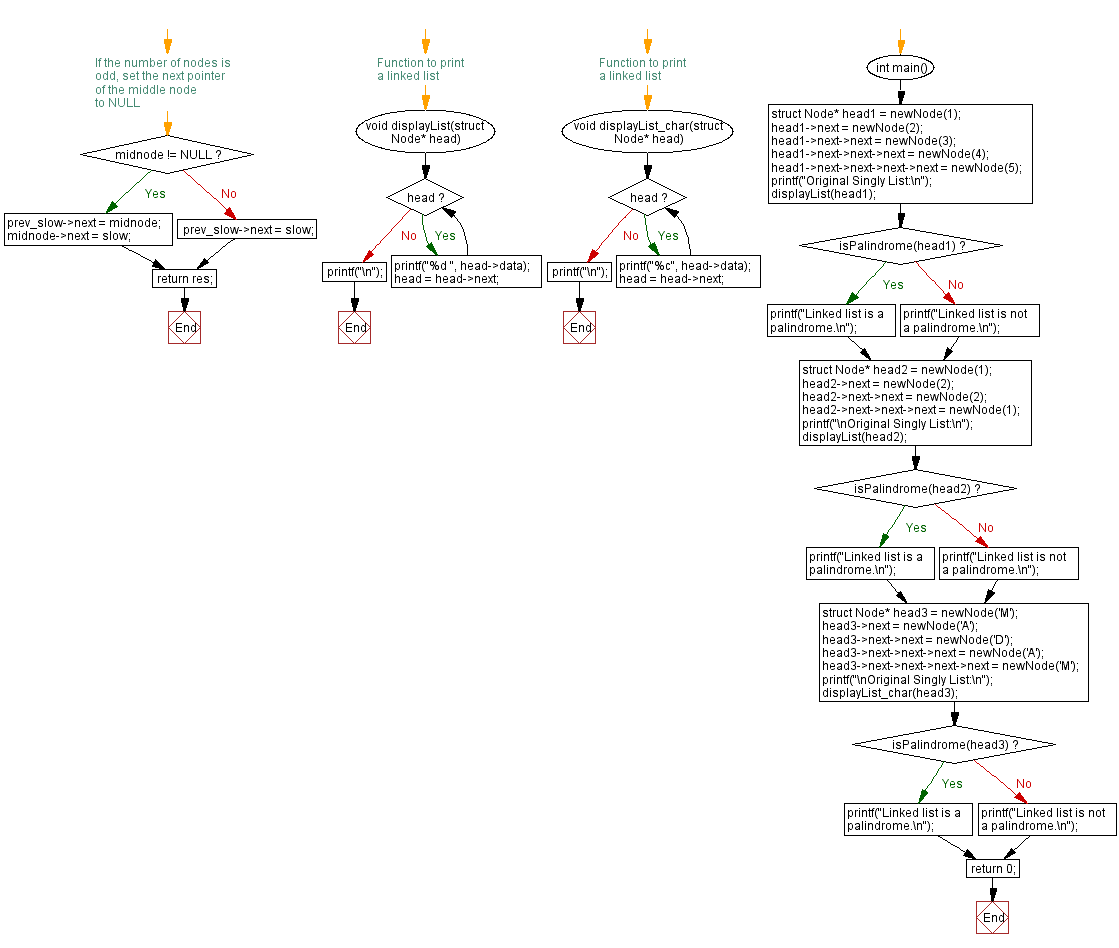﻿ C Program: Check if a singly linked list is palindrome or not

# C Exercises: Check if a singly linked list is palindrome or not

## C Singly Linked List : Exercise-15 with Solution

Write a C program to check if a singly linked list is a palindrome or not.

Sample Solution:

C Code:

``````#include<stdio.h>
#include <stdlib.h>

struct Node {
int data;
struct Node* next;
};

// Function to create a new node
struct Node* newNode(int data) {
struct Node* node = (struct Node*) malloc(sizeof(struct Node));
node->data = data;
node->next = NULL;
return node;
}

// Function to reverse a linked list
struct Node* reverseList(struct Node* head) {
struct Node* next = NULL;
struct Node* prev = NULL;

while (current) {
next = current->next;
current->next = prev;
prev = current;
current = next;
}

return prev;
}

// Function to check if a linked list is a palindrome
struct Node* midnode = NULL;
bool res = true;

// Find the middle node
while (fast && fast->next) {
fast = fast->next->next;
prev_slow = slow;
slow = slow->next;
}

// If the number of nodes is odd, skip the middle node
if (fast != NULL) {
midnode = slow;
slow = slow->next;
}

// Reverse the second half of the linked list
slow = reverseList(slow);

// Compare the first and second half of the linked list
while (slow != NULL) {
if (fast->data != slow->data) {
res = false;
break;
}

fast = fast->next;
slow = slow->next;
}

// Reverse the second half of the linked list again
slow = reverseList(slow);

// If the number of nodes is odd, set the next pointer of the middle node to NULL
if (midnode != NULL) {
prev_slow->next = midnode;
midnode->next = slow;
} else
prev_slow->next = slow;

return res;
}
// Function to print a linked list
}
printf("\n");
}

// Function to print a linked list
}
printf("\n");
}

int main() {
printf("Original Singly List:\n");
else
printf("Linked list is not a palindrome.\n");

printf("\nOriginal Singly List:\n");
else
printf("Linked list is not a palindrome.\n");

printf("\nOriginal Singly List:\n");
else
printf("Linked list is not a palindrome.\n");

return 0;
}
```
```

Sample Output:

```Original Singly List:
1 2 3 4 5
Linked list is not a palindrome.

Original Singly List:
1 2 2 1

Original Singly List:
```

Flowchart :C Programming Code Editor:

What is the difficulty level of this exercise?

﻿